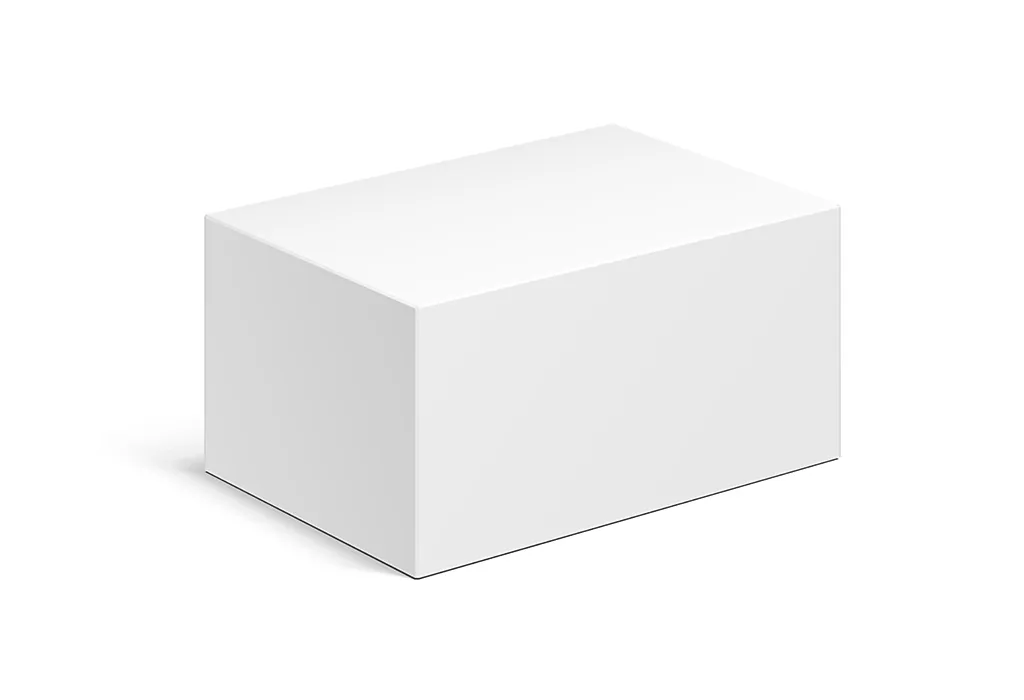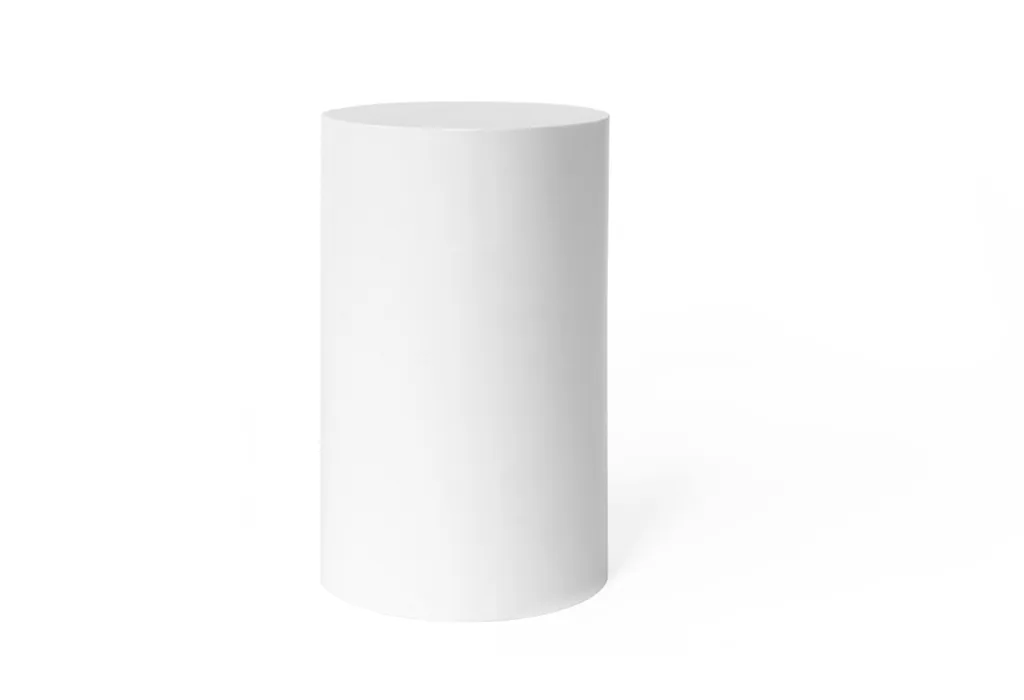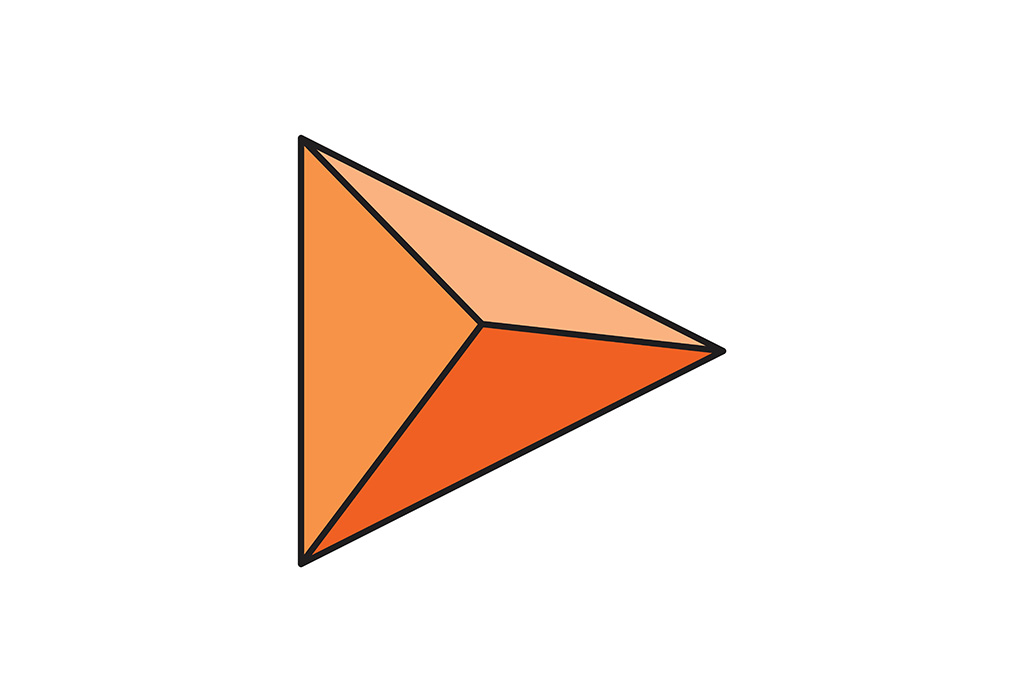Solid Shapes For Kids - Types And Examples

# Solid Shapes For Kids – Types And Examples

Learning about shapes is an essential part of a child’s early education. While most children are familiar with flat, two-dimensional shapes, introducing them to three-dimensional or solid shapes is an exciting journey into the world of geometry. This guide will take you through the various types of solid shapes for kids and offer some interesting examples to make the learning process fun.

## What Are Solid Shapes?

Solid shapes, often referred to as “solid shapes in maths,” are three-dimensional objects that have length, breadth, and height. Unlike two-dimensional shapes, which are flat and have only length and width, solid shapes have depth as well. They occupy space in the real world and can be touched, held, and moved around.

Children encounter many of these shapes in their daily lives. For instance, a soccer ball resembles a sphere, while a cereal box can be likened to a rectangular prism. The study of solid shapes in maths not only enhances spatial awareness but also builds the foundation for more advanced geometric concepts.

To help children grasp the idea, here are some common examples of solid shapes:

1. Sphere: Think of planets or basketballs.

2. Cuboid (Rectangular Prism): Like boxes or bricks.

3. Cube: The dice used in board games is a perfect example.

4. Cylinder: Soft drink cans and certain types of batteries.

5. Cone: Ice cream cones or traffic cones.

6. Pyramid: The ancient pyramids of Egypt or certain board game pieces.

Understanding these basic solid shapes lays the groundwork for kids to delve deeper into the world of geometry and to appreciate the myriad forms and structures they encounter in their surroundings.

## Different Types Of Solid Shapes

Solid shapes are three-dimensional figures that occupy space. They’re all around us, from the buildings we live in to the toys kids play with. While there are numerous solid shapes, we’ll be focusing on seven primary types. Each shape has its unique set of properties that defines it.

### 1. Cube#### Properties of Cube:

1. It has 6 identical square faces.

2. It has 12 equal edges.

3. All its angles are right angles.

4. It has 8 vertices (corners).

####### Properties of Cuboid:

1. It has 6 faces, where opposite faces are equal.

2. It has 12 edges.

3. All internal angles are right angles.

4. It has 8 vertices.

### 3. Sphere#### Properties of Sphere:

1. It has one continuous curved surface.

2. It does not have any edges or vertices.

3. All points on the surface are equidistant from its center.

4. It has a constant cross-section in any direction.

####### Properties of Cylinder:

1. It has 2 flat, parallel circle bases and one curved surface.

2. It does not have any vertices.

3. The cross-section perpendicular to its base gives a circle.

4. The length between the two bases is called the height.

### 5. Cone#### Properties of Cone:

1. It has 1 flat circular base and 1 curved surface.

2. It has a pointed vertex opposite its base.

3. The length from the base to the vertex is called the height.

4. The straight line joining the vertex and the midpoint of the base circle is the axis.

### 6. Pyramid#### Properties of Pyramid:

1. It has a polygon base and triangular faces that converge to a single point called the apex.

2. The number of triangular faces equals the number of sides in the base polygon.

3. The base can be any polygon, like a triangle, square, or hexagon.

4. All the triangular faces meet at the common vertex or apex.

####### Properties of Tetrahedron:

1. It is a pyramid with a triangular base.

2. It has 4 equilateral triangle faces.

3. It has 6 equal-length edges.

4. It has 4 vertices.

Understanding these properties can help in distinguishing between different solid shapes and provide a foundation for more complex geometric principles.

## What Are The Faces, Edges, And Vertices Of Solid Shapes?

Solid shapes are a significant part of geometry, with their properties and characteristics forming the basis of spatial understanding. An integral way to distinguish and understand solid shapes is by examining their faces, edges, and vertices. Each of these components offers insights into the nature, structure, and type of the 3D object in question. Let’s delve deeper into each of these aspects:

### Faces

• A face is a flat or curved surface on a solid shape. For instance, a cube has six flat faces, while a sphere has one curved face.

• The faces determine the shape and type of the 3D object. For example, a pyramid’s faces are triangular, leading to its distinctive structure.

### Edges

• Edges are the line segments where two faces of a solid shape meet. A cube, for example, has 12 edges.

• The number and length of edges can help in identifying the solid shape. A tetrahedron has 6 equal-length edges, distinguishing it from other pyramids.

### Vertices

• A vertex (plural: vertices) is a point where two or more edges meet. A cube has 8 vertices.

• The number of vertices can often indicate the type of solid shape. For instance, a tetrahedron has 4 vertices, while a rectangular prism or cuboid has 8.

Remembering these core components and their characteristics is vital when exploring solid shapes for class 1, 2, and 3 students, making their journey in the world of geometry smooth and enlightening.

## Important Points To Remember About Solid Shapes

Solid shapes form the basis of our understanding of the three-dimensional world around us. Whether it’s the structures we inhabit, the toys children play with, or the natural forms we see in nature, solid shapes are integral. Here are some crucial points to bear in mind when thinking about solid shapes:

1. Dimensionality: Unlike 2D shapes which have only length and width, solid shapes have a third dimension – depth. This is what gives them volume and makes them occupy space in the real world.

2. Uniformity is not mandatory: Solid shapes can have uniform faces and angles, like cubes, or they can have varied faces and angles, like pyramids. Their variety is vast, spanning regular shapes like the tetrahedron to irregular ones like certain crystals.

3. Real-world application: Solid shapes are foundational in multiple disciplines such as architecture, engineering, and design. Understanding their properties is essential for these applications. For instance, the strength of a cylindrical pillar versus a square one can determine architectural choices.

4. Surface and Volume: Every solid shape has both an external surface area and an internal volume. The surface area is the total area that the surface of the object occupies, while the volume represents the space enclosed by the shape. Both are essential metrics in various real-world scenarios, from packaging design to construction.

Remembering these key points can provide a solid foundation (pun intended!) for diving deeper into the world of geometry and appreciating the multifaceted nature of the 3D world around us.

## Solved Examples Of Solid Shapes For Children

Teaching kids about geometry can be a fun experience, especially when solid shapes are introduced. Practical, hands-on examples can make these concepts relatable and understandable. Here are some simple examples tailored for children in the age group of 5-8 years:

#### Example 1

Problem:
Emma has a toy box in the shape of a cube. All its sides are of the same length. If one side of the cube is 10 units long, how long is the opposite side?

Solution:
Since the toy box is a cube, all its sides are of equal length. So, the opposite side is also: = 10 units

#### Example 2

Problem:
Lucas is playing with a dice (which is a cube) and a toy pyramid. If the dice has 6 faces, how many faces does the toy pyramid have?

Solution:
A pyramid has one base and triangular faces that meet at the top. So, for a pyramid with a square base, it will have: = 1 base + 4 triangular faces = 5 faces

#### Example 3

Problem:
Liam has a toy in the shape of a cylinder. He notices it has two flat faces and one curved face. How many total faces does the cylinder have?

Solution:
Total number of faces in a cylinder: = 2 flat faces + 1 curved face = 3 faces

#### Example 4

Problem:
Sophia has 3 marbles, each in the shape of a sphere. If she looks at one marble, how many flat faces can she find?

Solution:
A sphere is a perfectly round solid shape. So, it has: = 0 flat faces

These hands-on, tangible solid shapes examples make it easier for children in the 5-8 years age group to visualize and understand the basic properties of three-dimensional shapes in a fun and engaging manner.

To test your child’s understanding and make learning interactive, here are some practice questions related to solid shapes for kids

Q1: Which solid shape has only one flat face and one curved face?

Choices:

a) Cube
b) Cone
c) Sphere
d) Pyramid

b) Cone

Q2: How many vertices does a cube have?

Choices:

a) 6
b) 8
c) 4
d) 12

b) 8

Q3: What shape is a basketball most similar to?

Choices:

a) Cylinder
b) Sphere
c) Cube
d) Prism